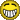# How can I expand a one-bit signal to multi bits?ClassicListThreaded2 messagesWhen I design ALU, for example there is a  one-bit input: ZX I want to use these logic to implement the :setting zero function: And16(a=x, b=zx, out=out1); Xor16(a=x, b=out1, out=outzx);    I firstly think it will work,because:  if zx=1, out1=x,   Xor(x,x)=0, if zx=0, out1=0, Xor(x,0)=x; but the simulator says : zx is one-bit. I want to expand it to 16bits. At last , I changed to use Mux16 to implement this.      further, I wonder  how to implement the "if ..  else.." in HDL ,except use Mux16.  thanks a lot!!                 I am green.